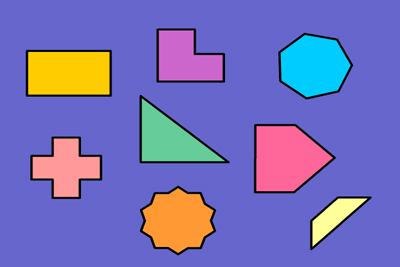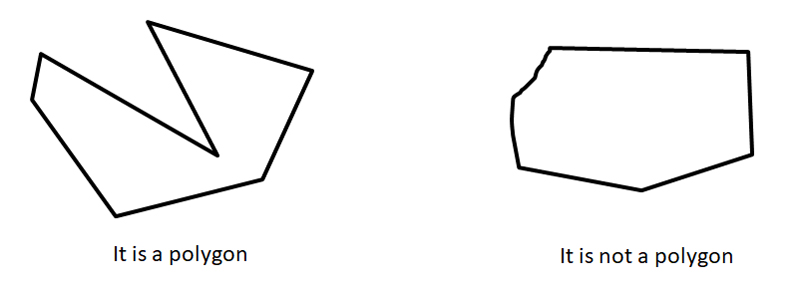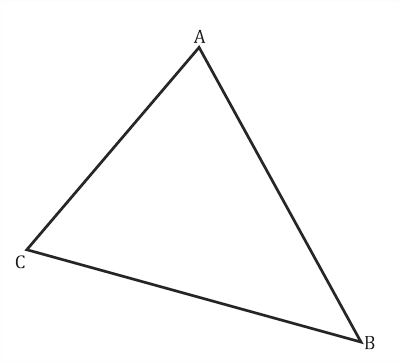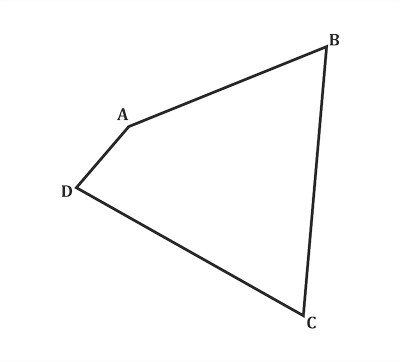Polygon - Geometry
28-06-2020    67 timesIf three or more line or line segment formed a closed shape, then it is known as a polygon. Consider all the sides of polygon are a line or line segments and each side of the line must intersect exactly two others sides but only at their endpoints. The sides of the polygon must be non-collinear and have a common endpoint. If one of the sides of a closed shape is a curve then it not consider as a polygon.All the geometric shapes are classified based on the number of sides.

• Triangle (3-sides): Triangles are a three-sided polygon that consists of three edges and three vertices.

Here Three Sides are AB, BC, CA.
Here three vertices are ∠ABC, ∠BCA, ∠CAB.• Quadrilateral (4-sides): A polygon or 2D geometric shape has four sides or edges and four corners or vertices.

Here four sides are AB, BC, CD, DA.
Here four vertices are ∠ABC, ∠BCD, ∠CDA, ∠DAB.• Pentagon (5-sides)
• Hexagon (6-sides)
• Heptagon (7-sides)
• Octagon (8-sides) and so on.